[Contents]

Basic Properties of Differential Forms
John Denker

## 1  Definitions and Basic Properties

We define differential forms to have the following properties:

1.    We assume the existence of a space with coordinates x1, x2, ⋯. In thermodynamics we might choose the coordinates to be V, S, ⋯. You can choose almost any coordinates you like; the approach outlined here works for any set of coordinates, assuming they are nonsingular, along with a few other mild restrictions. The coordinates do not need to be mutually perpendicular; see item 15.

2.    For each i, we postulate the existence of something denoted [dxi] and call it a differential form. (We shall soon prove that we can do without the square brackets, but for the moment they are part of the definition.)

3.    Every differential form has a grade. The forms just mentioned have grade=1. They are called 1-forms for short.

4.    A plain old scalar is considered a grade=0 form. This includes scalar-valued functions f(x1, x2, ⋯).

5.    In simple cases at least, you may interpret a 1-form as measuring a slope. For example, [dx1] represents something with unit slope, sloping up in the x1 direction, as exemplified in figure 1. A good example of a more complicated 1-form is geographic slope, as depicted by the contour lines on a topographic map. Closely-spaced contour lines represent a steep slope. See item 10 for more on this.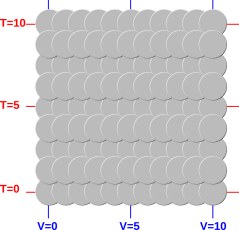Figure 1: A Very Simple One-Form

6.    The most general 1-form is a linear combination of other 1-forms, such as

 B = f(x1, x2, ⋯) [dx1] + g(x1, x2, ⋯) [dx2] + ⋯
(1)

for arbitrary scalar-valued functions f, g, et cetera. So we are using the set {[dxi]} as a basis.

7.    The alert reader may have noticed that forms have a magnitude and direction, and they behave just like vectors. That’s true and important. If you define the notion of “vector” properly, one-forms are definitely vectors. However it is crucial to keep in mind the distinction:

 There exist pointy vectors, which are relatively familiar to most people. They can be represented by an arrow with a tip and a tail. There exist one-forms, which are relatively less familiar to most people. They can be represented by contour-lines, or by stacks of fish-scales as in figure 1.

Pointy vectors and one-forms show up in quite a variety of contexts. They are given a variety of different names, and can be visualized in a variety of different ways, as tabulated near the end of item 10.

As we shall see, pointy vectors and one-forms have quite a few properties in common, since they all obey the vector-space axioms. On the other hand, there are also some crucial differences between pointy vectors and one-forms, so we have to be careful. Item 12 discusses one of the differences you need to watch out for.

8.    We define the exterior derivative operator d applied to a scalar function as follows, in terms of our basis set {[dxi]} and the chain rule:

df(x1x2, ⋯) =
 ∑ i
[dxi
 ∂ f ∂ xi

 {all xj except xi}
(2)

where in the ith term of the sum, the partial derivative holds constant all the arguments to f() except for the xi argument. The notation for this is clumsy, but the idea is important. The partial derivative is really a directional derivative in a direction specified by holding constant an entire set of variables except for one … so it is crucial to know the entire set, not just the one variable that is nominally being differentiated with respect to. For details on this, including ways to visualize what it means, see reference 1.

An example is shown in figure 2. The intensity of the shading depicts the height of the function F := sin(x1)sin(x2) while the contour-lines depict the exterior derivative dF.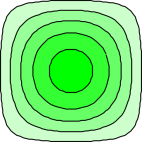Figure 2: A Function and its Exterior Derivative

9.    If you choose f(x1, x2, ⋯) = x1 in equation 2, you can easily prove that

 dx1 = [dx1]
(3)

which is convenient. It simplifies the notation.

Technically speaking, [dx1] exists by fiat, according to item 2, while dx1 is something you can calculate according to equation 2. On a day-to-day basis you don’t care about the distinction, but it would have been cheating to assume they are equal. We needed to keep them conceptually distinct just long enough to prove they are numerically equal.

10.    You can visualize a pointy-vector as a little arrow with a “tip” and a “tail”, but you should not visualize a 1-form the same way.

Suppose we want to visualize the gradient of some landscape. If you visualize the gradient as a pointy vector, it points uphill. In many cases, though, you are better off visualizing the gradient as a one-form, corresponding to contour lines that run across the slope.

You can judge the magnitude of the 1-form according to how closely packed the contour lines are. Closely-packed contours represent a large-magnitude 1-form. To say the same thing the other way, the spacing between contours is inversely related to the magnitude of the one-form.

Contour lines have the wonderful property that they behave properly under a change of coordinates: if you take a landscape such as the one in figure 2 and stretch it horizontally (keeping the altitudes the same) as shown in figure 3, the slopes become less. The contour lines on the corresponding topographic map spread out by the same stretch factor, as they should, to represent the lesser slope. In contrast, if you try to represent the gradient by pointy vectors, the representation is completely broken by a change in coordinates. As you stretch the map, position vectors and displacement vectors get longer, but gradient vectors have to shorter, to represent the lesser slope. Therefore we need different representations. We represent positions and displacements using pointy vectors, but we represent gradients using 1-forms.

To say the same thing the other way, representing a gradient using a pointy vector would be a bad idea; such vectors would not behave properly. They would not be “attached” to the landscape the way contour lines are.Figure 3: Stretching a Coordinate Increases the Distance and Decreases the Steepness

Of course, pointy vectors are needed also; they are appropriate for representing the location of one point relative to another in this landscape. These location vectors do stretch as they should when we stretch the map. An example of this is shown in red in figure 3.

It is important to clearly distinguish the two types of vector. The distinction shows up in a variety of different contexts:

 Type of vector: pointy vector one-form In linear algebra: column vector row vector In relativity: contravariant vector covariant vector In quantum mechanics: Dirac ket:  |⋯⟩ Dirac bra:  ⟨⋯| On a map: displacement gradient Stretching the map: increases distance decreases steepness

If not all the rows in that table are familiar to you, don’t worry about it.

11.    Some remarks about the terminology:
• The difference between two position-vectors is called a separation-vector or equivalently a displacement-vector.
• A position-vector depends on the choice of origin, but a displacement-vector does not.
• The magnitude of a displacement-vector is called the distance. The distance is a scalar, not a vector.
• The magnitude of a gradient-vector is called the steepness.
• In one dimension, and sometimes even in higher dimensions, a gradient may be called a slope.

12.

Consider the contrast:

 In some spaces, we have a metric. That is, we have a dot product. That allows us to determine the length of a vector, and to determine the angle between two vectors. In such a space, we have a geometry (not just a topology). Ordinary Cartesian (x,y,z) space is a familiar example. There are other spaces where we do not have a metric. We do not have a dot product. We do not have any notion of angle, and not much notion of length or distance. We have a topology, but not a geometry. Thermodynamic state-space is an important example. We can measure the distance (in units of S) between contours of constant S, but we cannot compare that to any distance in any other direction.

 If we have a metric, we can use it to transpose a vector. Transposing a row vector creates the corresponding column vector and vice versa. That gives us a one-to-one correspondence: For any pointy vector you can find a corresponding one-form and vice versa. In a non-metric space, there is not any way of converting 1-forms to pointy vectors or vice versa. There is not any way of finding a 1-form that uniquely “corresponds” to a given pointy vector or vice versa.

 Technically, the row vectors always live in their own space, while the column vectors always live in another space.

 Given a metric, the two spaces are isomorphic, and people usually don’t bother to distinguish them. Without a metric, the two spaces remain distinct. It makes sense to visualize dE as a one-form, i.e. as contours of constant E ... but it does not make sense to visualize dE as any kind of pointy vector. It makes even less sense to visualize dE as any kind of scalar, infinitesimal or otherwise. E is a scalar, but the gradient of E (i.e. dE) is a one-form.

13.    Note that when we stretch the map, as in figure 3, the following topological property is preserved: The red arrow crosses three contours. The “number of crossings” is three, both before and after the stretch. After the stretch, the steepness is less but the distance is greater. More precisely, the product between the gradient-vector and the separation-vector is unchanged. (This type of product is called a contraction, as discussed in section 2.2.) This is related to the fact that in this example, height is a function of state. Height is unchanged by horizontal stretching. The tip of the red arrow is three units higher than the tail, both before and after the stretch.

14.    In three dimensions, rather than having contour “lines” we have contour “shells”, like the layers of an onion. If T is the temperature in the room, you can visualize dT as shells, each shell representing a constant temperature. More generally, in D dimensions, the contours are objects with dimensionality D−1.

15.    There are many situations – including thermodynamics – where it is not possible to define any notion of length or angle. To say the same thing in mathematical terms, it is not possible to define a dot product.

A 1-form has a direction, but we cannot measure the angle between two such directions. You can say that we have a topology but not a geometry. This sounds like a terrible limitation, but it is actually the right thing for thermodynamics, because typically you have no way of knowing whether dS is “perpendicular” to dV or not, and it causes all sorts of trouble if you use a mathematical formalism that assumes you can measure angles when you can’t.

Among other things, this means that we do not require the coordinates x1, x2, ⋯ to be mutually perpendicular. Since there is no notion of distance or angle, so we could not make them perpendicular even if we wanted to. See figure 4.Figure 4: Contours of Constant Value (for three different variables)

16.    The wedge product of two 1-forms is written dxidxj and is a grade=2 differential form, called a 2-form for short.

17.    The wedge product is associative: A ∧ (BC) = (AB) ∧ C. This means we can take the wedge product of forms of any grade without worrying about parentheses.

18.    The wedge product between grade=1 forms is antisymmetric:

 dxi ∧ dxj = −dxj ∧ dxi              (4)

for all (i, j).

19.    As a consequence of the foregoing, the wedge product between an odd-grade form and an even-grade form is symmetric: A ∧ dx1 = dx1A if A is a scalar or a 2-form, even though it is antisymmetric if A is a 1-form. For this reason, it is OK to omit the wedge symbol when multiplying something by a scalar, as in equation 1.

20.    A differential form F is called grady if it is the exterior derivative of some other form: F = dφ. A good example from thermodynamics is the form PdV + VdP, which is grady because it equals d(PV). In contrast, PdV by itself is not grady.

Non-grady force fields are common in the real world. See reference 2 for more about how to visualize such things.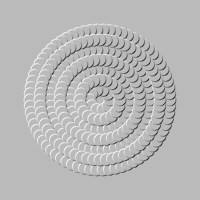Figure 5: A One-Form that is Not Grady

A conspicuously ungrady form w is shown in figure 5. You can imagine that this represents the 1-form w := PdV (aka “work”) in a slightly-idealized heat engine. The direction of the 1-form (i.e. the uphill direction) is everywhere counterclockwise. This w is a perfectly fine 1-form, but you cannot write w = dW because w cannot be the slope of any potential W. The concept of slope is locally well-defined, and you can integrate the slope along a particular path from A to B, but you cannot use this integral to define a potential difference W(B) − W(A) because the result depends very much on which path you choose. This is like Escher’s famous “Waterfall” shown in figure 6.Figure 6: Waterfall, by M. C. Escher (1961) ./img48escher-waterfall.jpg

To repeat: You are free to write w = PdV. That is a perfectly fine 1-form, well-defined at every point in the state space. In contrast, it is not OK to write w = dW or PdV = dW, because that cannot be well-defined throughout state space, except perhaps in trivial cases. (You might be able to define something like that on a one-dimensional subspace, along a particular path through the system, but then you would need to decorate “W” with all sorts of subscripts to indicate exactly which subspace you are talking about.)

A more subtle example of an ungrady form is discussed in item 23 below.

21.    The exterior derivative d applied to a grade=1 or higher object obeys a product rule (as you would expect for a derivative) with anti-symmetry (as you would expect for a wedge product), namely

 d(A ∧ B) = dA ∧ B + (−1)k A ∧ dB              (5)

22.    As a consequence of the definition in item 21, it turns out that the operator d applied twice to any potential f is necessarily zero. That is ddf≡0 for any f. Since f doesn’t matter, we can drop it and write the elegant operator equation:

 dd = 0              (6)

This important result can be expressed in words: “the boundary of any boundary is zero”.

Before we explain why this is so, we should emphasize that dd is not the most general second-derivative operator. Rather, it is the antisymmetric part of the second derivative, in accordance with equation 5. So what we are saying is that the antisymmetric part of the second derivative vanishes.

• By way of contrast, the symmetric part would be the familiar Laplacian ∇·∇, but that only makes sense if we have a well-defined dot product, which is not generally the case in thermodynamics.
• As another type of contrast, d applied to a scalar function is the most general first derivative, namely the gradient, even though dd is not the most general second derivative.

The antisymmetric piece of the second derivative necessarily vanishes, because of the mathematically-guaranteed symmetry of mixed partial derivatives:

 ∂ ∂x|y
 ∂ ∂y|x
f  ≡
 ∂ ∂y|x
 ∂ ∂x|y
f              (7)

This is true for all f, assuming f is sufficiently differentiable, so that the indicated derivatives exist.

Figure 7 and Figure 8 show what’s going on. We will use these figures to discuss finite steps (as indicated by Δ) instead of infinitesimals, but the same ideas apply in the limit of very small steps. In particular, Δx|y means to take a step toward increasing x along a contour of constant y. Similarly Δy|x means to take a step toward increasing y along a contour of constant x.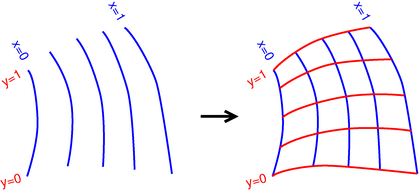Figure 7: Boundary of a Boundary: X then Y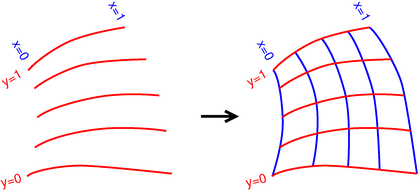Figure 8: Boundary of a Boundary: Y then X

In accordance with the usual operator-precedence rules, the interpretation of the LHS of equation 7 is:

 ∂ ∂x|y

 ∂ ∂y|x
f      means

 ∂ ∂x|y

 ∂ ∂y|x
(f)

(8)

That is, we work from right to left, first taking a step toward increasing y along a contour of constant x, then taking a step toward increasing x along a contour of constant y. For example, in the figure, this would correspond to proceeding clockwise from (0,0) via (0,1) to (1,1).

Meanwhile, the RHS of equation 7 tells us to proceed counterclockwise in the figure, from (0,0) via (1,0) to (1,1). The point is that we get to same point either way. That is, the clockwise trip we just took, together with the counterclockwise trip, form a “closed” figure.

This result is nontrivial. Although the boundary of a boundary is zero, the boundary of “something else” is not necessarily zero. For example:

 d(dS) = 0 but d(TdS) = dT∧dS + T ddS = dT∧dS = not necessarily 0
(9)

23.    A form F is called closed if its exterior derivative dF vanishes. By equation 6, we know that every grady form is closed, but the converse does not hold. In a universe with periodic boundary conditions, such as the cylinder shown in figure 9, you can have a closed one-form that is not grady. The form points everywhere counterclockwise as you go around the universe. Rather than fish-scales, in this figure we use color-coded “contour” lines. The “contour” lines are shown with solid blue on their positive (“uphill”) side and dashed red on their negative (“downhill”) side. The form is in fact a constant everywhere on the cylinder, so it satisfies the boundary conditions and satisfies dF=0.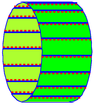Figure 9: A Form that is Closed but Not Grady

Forms that are closed, including figure 2 and figure 9, have the property that the “contour” lines in one region mesh nicely with the lines in adjacent regions. In a non-closed form such as figure 5, the meshing fails somewhere. (Commonly it fails everywhere.)

Beware that this notion of “closed one-form” is not equivalent to the notion of “closed set” (containing its limit points) nor to the notion of “closed manifold” (compact without boundary). See reference 3 and reference 4.

24.    We want to be able to integrate our differential forms. For a grady form, this is easy. We postulate that

 B A
dF = F(B) − F(A)              (10)

The meaning is simple: the integral measures the number of contours that you cross in going from point A to point B. For a grady 1-form, this number is independent of the path you take along the way from A to B.

This integral is, of course, a linear operator.

25.    To integrate an ungrady 1-form, we need to specify the path. Let the general point on the path C be denoted C(θ), where θ is a parameter that varies smoothly and monotonically as we progress along the path. Recall that the general 1-form B can be written as a superposition:

 B = fi(x) dxi              (11)

We are using the Einstein summation convention, i.e. implied summation over repeated indices, such as index i in this equation.

As explained in section 2, the integral of this is:

 along C
B
 θ(end) θ(start)
fi(C(θ))
 ∂ Ci ∂ θ
dθ

(12)

## 2  Integrating a One-Form

### 2.1  Explanation

To understand how we integrate a one-form B along the curve C, start by breaking the curve into small segments and integrating each segment separately:

 along C
B
 along C
fi(xdxi
=
 C(θ2) C(θ1)
fi(xdxi
+
 C(θ3) C(θ2)
fi(xdxi
+
 C(θ4) C(θ3)
fi(xdxi
+
(13)

and if f is a sufficiently smooth function and if C is a sufficiently smooth curve, and if the points {θ1, θ2, ⋯} are sufficiently close together, then we can treat f as being locally constant and pull it out front of the integrals:

 along C
B
 along C
fi(xdxi
=
fi(C(θ1))
 C(θ2) C(θ1)
dxi
+
fi(C(θ2))
 C(θ3) C(θ2)
dxi
+
fi(C(θ3))
 C(θ4) C(θ3)
dxi
+
(14)

Now we have grady forms inside the integral, so we can integrate them immediately using equation 10. We get

 along C
B
 along C
fi(xdxi
= fi(C(θ1)) [Ci(θ2) − Ci(θ1)]
+ fi(C(θ2)) [Ci(θ3) − Ci(θ2)]
+ fi(C(θ3)) [Ci(θ4) − Ci(θ3)]
+
(15)

where we have described the point C(θ) using an expansion in terms of the basis vectors:

 C(θ) = Ci(θ) xi              (16)

Equation 15 is beginning to look like a familiar Riemann integral. In fact it is just

 along C
B
 along C
fi(xdxi
 θ(end) θ(start)
fi(C(θ))
 ∂ Ci ∂ θ
dθ

(17)

### 2.2  No Dot Product

In equation 17, do not think of the integrand as a dot product, even though it involves the same sum-of-products you would use for evaluating f · ∂C/∂θ. We do not have a dot product. The operation here is a contraction. A contraction involves a one-form acting on a pointy vector. In this case the one-form is f and the pointy vector is ∂C/∂θ. In equation 15, you can visualize [Ci(θ2) − Ci(θ1)] as a pointy vector with its tip at C(θ2) and its tail at C(θ1).

We can carry out the contraction of a one-form with a pointy vector. We cannot carry out the dot product of two one-forms, nor the dot product of two pointy vectors. Think of one-forms as 1×D matrices (one row and D columns) and pointy vectors as D×1 matrices.

### 2.3  Example

As an example, consider integrating the one-form

f :=
 −x2 r
dx1 +
 x1 r
dx2              (18)

where r := √(x12 + x22). This one-form is depicted, with fair accuracy, in figure 5. We wish to integrate it along a curve C which is a circular path of radius R, centered on the origin, so that along C:

 x1 = R cos(θ) x2 = R sin(θ) ∂C1/∂θ = −R sin(θ) ∂C2/∂θ = R cos(θ)
(19)

Plugging in to equation 17 we find

 along C
f
=
R sin2(θ) + R cos2(θ)dθ
= R
(20)

## 3  Applications

For a discussion of how differential forms apply to thermodynamics, see reference 5.

## 4  References

1.
John Denker,
“Partial Derivatives – Pictorial Interpretation”
www.av8n.com/physics/partial-derivative.htm
2.
John Denker,
“Visualizing A Field that is Not the Gradient of Any Potential”
3.
Mathworld entry: “Closed Set”
http://mathworld.wolfram.com/ClosedSet.html
4.
Mathworld entry: “Closed Manifold”
http://mathworld.wolfram.com/ClosedManifold.html

(beware: at some points this assumes the existence of a dot product.)

5.
John Denker,
“Thermodynamics and Differential Forms”
www.av8n.com/physics/thermo-forms.htm
[Contents]